## FISHEYE

 Applies a fisheye distortion to an image.

 USAGE: fisheye [-i ifov] [-o ofov] [-t type] [-f format] [-c xc,yc] [-r radius] [-a angle] [-m vpmethod] [-v vpcolor] [-b bgcolor] infile outfile USAGE: fisheye [-h or -help] -i .... ifov ......... input (perspective) image field of view in degrees; ...................... float; 00; default=min(width,height)/2 -a .... angle ........ angle of rotation (clockwise) for the fisheye image; ...................... float; 0<=angle<360; default=0 -m .... vpmethod ..... vp is the virtual-pixel method; default=black -v .... vpcolor ...... vpcolor is the color for the case when ...................... -virtual-pixel=background; this is where parts ...................... of the fisheye circle map outside image; ...................... use none for transparent; default=black -b .... bgcolor ...... bgcolor is the color for the area outside the ...................... circle of the fisheye area; ...................... use none for transparent; default=black PURPOSE: To apply a fisheye distortion to an image. DESCRIPTION: FISHEYE is designed to transform a normal perspective image into a fisheye view. ARGUMENTS: -i ifov ... IFOV is the input perspective image field of view in degrees. Values are floats in the range 0

### EXAMPLES

 Mandril (Square) Image - Fisheye Types / Circular OFOV=180 and IFOV=120 Original Image Linear Arguments: -o 180 -i 120 -t linear -f circular -m gray Equal Area Arguments: -o 180 -i 120 -t equalarea -f circular -m gray Orthographic Arguments: -o 180 -i 120 -t orthographic -f circular -m gray Stereographic Arguments: -o 180 -i 120 -t stereographic -f circular -m gray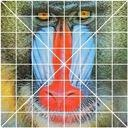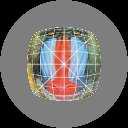Mandril (Square) Image - Fisheye Types / Circular OFOV=180 and IFOV=90 Original Image Linear Arguments: -o 180 -i 90 -t linear -f circular -m gray Equal Area Arguments: -o 180 -i 90 -t equalarea -f circular -m gray Orthographic Arguments: -o 180 -i 90 -t orthographic -f circular -m gray Stereographic Arguments: -o 180 -i 90 -t stereographic -f circular -m gray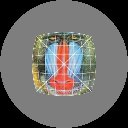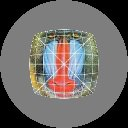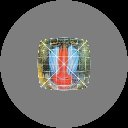Mandril (Square) Image - Fisheye Types / Full Frame OFOV=180 and IFOV=120 Original Image Linear Arguments: -o 180 -i 120 -t linear -f circular -m gray Equal Area Arguments: -o 180 -i 120 -t equalarea -f circular -m gray Orthographic Arguments: -o 180 -i 120 -t orthographic -f circular -m gray Stereographic Arguments: -o 180 -i 120 -t stereographic -f circular -m gray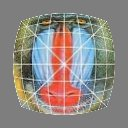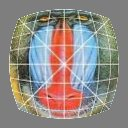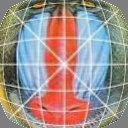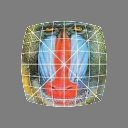Mandril (Square) Image - Fisheye Types / Circular Variations In Parameters Original Image Change Radius Arguments: -o 180 -i 120 -t linear -f circular -r 48 -m gray Change Angle Arguments: -o 180 -i 120 -t linear -f circular -a 90 -m gray Change Center Arguments: -o 180 -i 120 -t linear -f circular -c 48,64.5 -m gray Transparent Overlaid On Checkerboard Arguments: -o 180 -i 120 -t linear -f circular -m backround -v none -b none (post-processed to composite onto checkerboard background)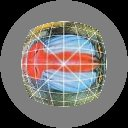Mandril (Square) Image - Fisheye Types / Circular Change In Distortion Original Image Arguments: -o 60 -i 60 -t linear -f circular -m gray Arguments: -o 90 -i 90 -t linear -f circular -m gray Arguments: -o 120 -i 120 -t linear -f circular -m gray Arguments: -o 150 -i 150 -t linear -f circular -m gray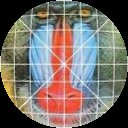Woman (Non-Square Image) - Linear - Circular vs. Fullframe Original Image Linear Circular Arguments: -o 180 -i 120 -t linear -f circular -m gray Linear Full Frame Arguments: -o 180 -i 120 -t linear -f fullframe -m gray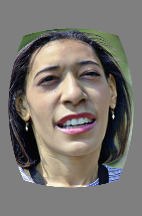What the script does is as follows: For a linear circular fisheye distortion, it applies the following equations: perspective: r=f*tan(phi); f=(N/2)/tan((fov/2)*(pi/180)) fisheye: r=f*phi; f=(N/2)/((fov/2)*(pi/180)) where N=smaller dimension This is equivalent to the following IM commands for the case of a perspective image of \${dim}x\${dim} to a linear circular fisheye image: compute image dimensions and center coordinates, etc xd="xd=i-\$xc;" yd="yd=j-\$yc;" rd="rd=hypot(xd,yd);" ofocinv=`convert xc: -format "%[fx:(\$ofov*pi)/(\$dim*180)]" info:` phi="phi=\$ofocinv*rd;" rr="rr=\$ifoc*tan(phi);" xs="xs=(rd?rr/rd:0)*xd+\$xc;" ys="ys=(rd?rr/rd:0)*yd+\$yc;" convert \$infile -virtual-pixel black -fx \ "\$xd \$yd \$rd \$phi \$rr \$xs \$ys (rd>\$dim/2)?black:u.p{xs,ys}" \ \$outfile